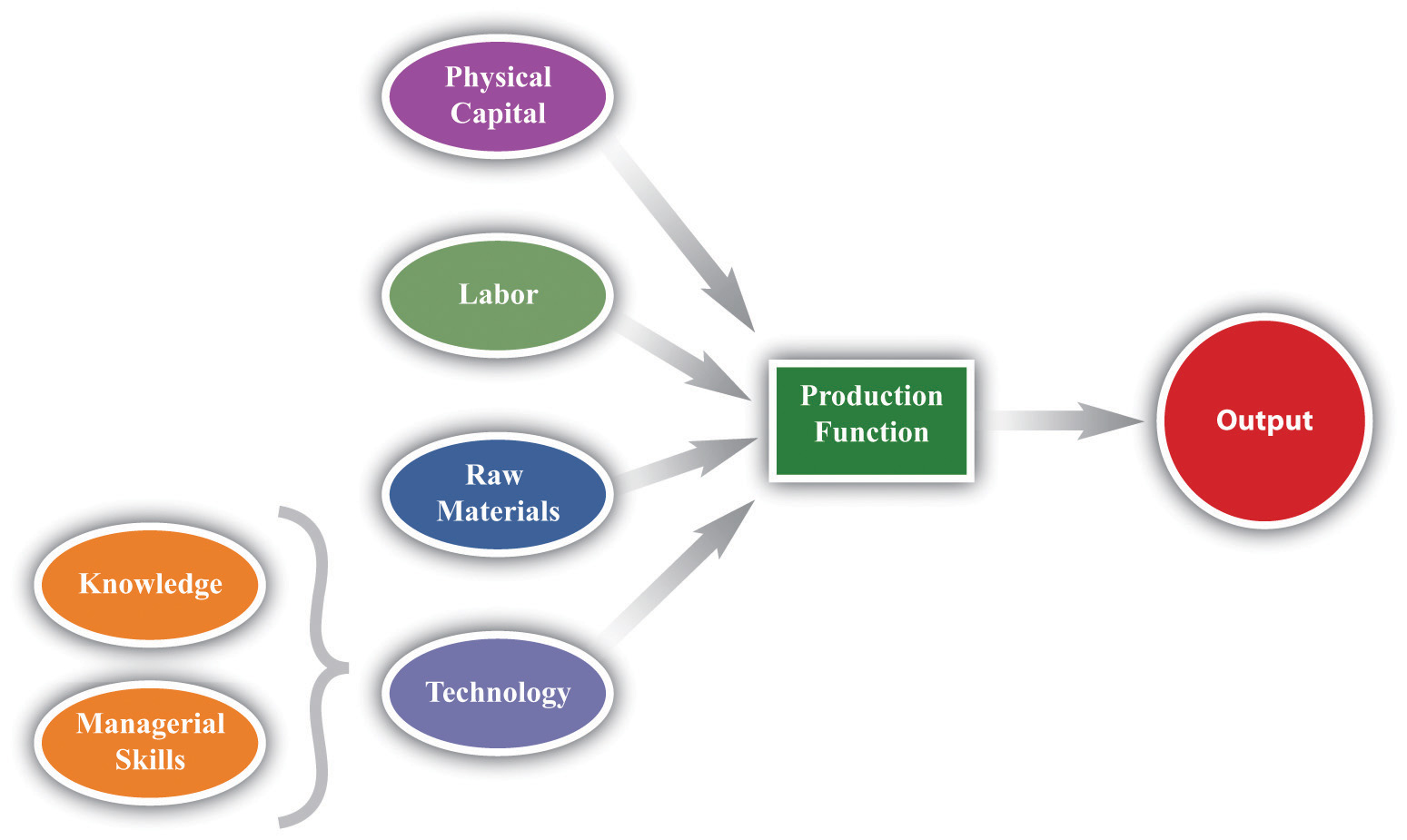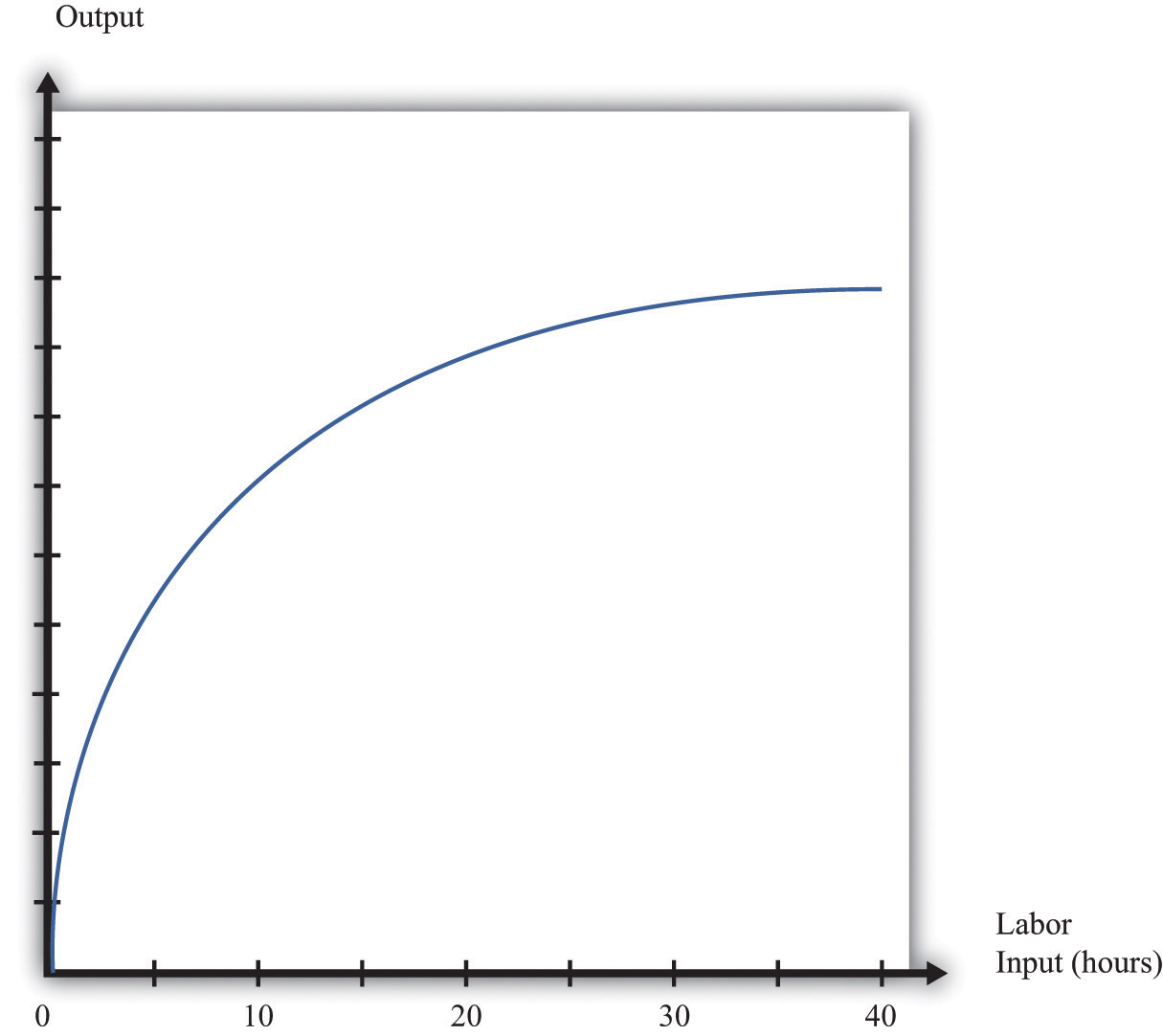This is “Production Function”, section 31.17 from the book Theory and Applications of Economics (v. 1.0). For details on it (including licensing), click here.

Has this book helped you? Consider passing it on:
Creative Commons supports free culture from music to education. Their licenses helped make this book available to you.
DonorsChoose.org helps people like you help teachers fund their classroom projects, from art supplies to books to calculators.

## 31.17 Production Function

The production function characterizes the output of a firm given the inputs it uses. The link between inputs and output is shown Figure 31.15 "The Production Function". The production function combines a firm’s physical capital stock, labor, raw materials (or intermediate inputs), and technology to produce output. Technology is the knowledge (the “blueprints”) that the firm possesses, together with managerial skills.

Production functions generally have two important properties:

1. Positive marginal product of an input
2. Diminishing marginal product of an input

By input, we mean any of the factors of production, such as physical capital, labor, or raw materials. The marginal product of an input is the extra output obtained if extra input is used. In this conceptual exercise, all other inputs are held fixed so that we change only one input at a time.

The first property asserts that additional output will be obtained from additional units of an input. Adding another machine, another worker, some more fuel, and so on, increases the output of a firm. A positive marginal product does not necessarily mean that the extra output is profitable: it might be that the cost of the extra input is high relative to the value of the additional output obtained.

The second property explains how the marginal product of an input changes as we increase the amount of that input, keeping the quantities of other inputs fixed. An additional unit of an input will (usually) increase output more when there is a small (rather than a large) amount of that input being used. For example, the extra output obtained from adding the first machine is greater than the additional output obtained from adding the 50th machine.

A simple production function relating output to labor input is shown in Figure 31.16 "Labor Input in the Production Function". This figure illustrates the two properties of positive and diminishing marginal product of labor. As more labor is added, output increases: there is a positive marginal product of labor (that is, the slope of the relationship is positive). But the extra output obtained from adding labor is greater when the labor input is low: there is diminishing marginal product of labor. From the graph, the slope of the production function (which is the marginal product of labor) is greater at low levels of the labor input.

## Key Insights

• The production function shows the output produced by a firm given its inputs.
• The production function displays two important properties: positive marginal product and diminishing marginal product.

Figure 31.15 The Production FunctionFigure 31.16 Labor Input in the Production Function